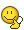## Recommended PostsNothing I do ever works. I made my solenoid type thing with about 300 turns, and Im using strong neodymium magnets... I get 0.02 volts when I pull the magnet out very fast... it is not enough.

I want to make a series of these solenoids so when I move the magnet across I want to "feel" a drag, and I want to actually produce some electricity (it will be AC but it's okay). If I move the magnet at 20-30m/s I want to get at least a few volts.

So what's the trick to making one of these generators? Am I building it wrong? What sort of resistors can I hook up so that I feel it hard in my hand to swipe the magnet across the top or the solenoids?##### Share on other sites

Since this would produce a very sharp spike, I am guessing that your voltmeter just isn't fast enough to capture it and is averaging it out over a larger time resolution.

##### Share on other sites

How can I calculate the maximum potential generated? I don't know how to calculate the field, all I have is the magnet but how do I measure its field in Tesla or Gauss?

##### Share on other sites
If I move the magnet at 20-30m/s I want to get at least a few volts.

Do you realise that 20 to 30m/s is 72 to 108km/h? I would think that is a little fast to do by hand.

How can I calculate the maximum potential generated? I don't know how to calculate the field, all I have is the magnet but how do I measure its field in Tesla or Gauss?

Well, you can use EMF(peak) = NBAw to work out the peak voltage produced. Where, N = number of turn of wire (coils)

B = magnetic field strength in Tesla

A = total internal area of the coils

w (which is meant to be a small omega) = angular momentum [ = 2(pi)*frequency]

But as you said you don't know the field strength...so, you could use F = BIl to work out an approximate B (field strength), from known force, current and length of wire. This might explain what to do.

## Create an account

Register a new account